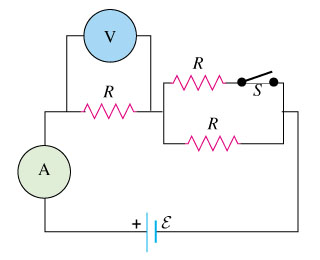# Problem: When the switch S in the figure is closed, the reading of the voltmeter V willa. increase.b. decrease.c stay the same.

###### FREE Expert Solution

From Ohm's law:

$\overline{){\mathbf{V}}{\mathbf{=}}{\mathbf{i}}{\mathbf{R}}}$, where V is voltage, i is current, and R is resistance.

91% (288 ratings)###### Problem Details

When the switch S in the figure is closed, the reading of the voltmeter V willa. increase.

b. decrease.

c stay the same.

Frequently Asked Questions

What scientific concept do you need to know in order to solve this problem?

Our tutors have indicated that to solve this problem you will need to apply the Resistors and Ohm's Law concept. You can view video lessons to learn Resistors and Ohm's Law. Or if you need more Resistors and Ohm's Law practice, you can also practice Resistors and Ohm's Law practice problems.